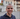# React.useMemo() for Optimisation

August 11, 2019

React.useMemo() is another method from the Hook API that helps to optimize performance. It memoizes and returns the same value for the expensive calculations if dependencies remain the same. For example, if you have a computational function inside the component, and that function receives props as arguments to calculate the value, usually you would like to spare the calculation if your component did update, but the props passed to function remained the same.

## How to Use It

The method has the following structure `React.useMemo(() => fn, deps)`. It takes two arguments: an inline function and an array of dependencies. Inline function, the first agument, returns a value from the expensive computation - `() => doHeavyComputation(a, b)`. The second argument is an array of dependencies `[a, b]`, when one of the dependencies changes, `useMemo()` recomputes the value, if it doesn’t - returns last memoized value.

``const memoisedValue = React.useMemo(() => doHeavyComputation(a, b), [a, b])``

If you forget to pass an array of dependencies, the new value computes every time the component renders.

Also important to note, that computational functions should be pure, without side effects. If you want to change the state in, for example, `doHeavyComputation()`, you should use `React.useEffect()` hook.

## When to Use It

React documentation states, that you should use it for expensive calculations. Here are a few examples where you might put it to use:

#### Filtering Large Arrays

``````function MyList(list, query) {
// On every component render it will be refiltered
const filteredList = filterListByQyery(list, query);

// Will recalculate only when the list or the query changes
const memoizedFilteredList = React.useMemo(
() => filterListByQyery(list, query),
[list, query],
);
}``````

#### Recursive functions, like Fibonacci series, factorial, etc.

``````function MySequance(number) {
// Will recalculate only when the number changes
const memoizedSequance = React.useMemo(() => getFibonacci(number), [number]);
}``````

#### Avoid unnecessary child component rendering

``````function Parent(a, b) {
// Will skip rerendering if the argument `a` will not change
const childA = React.useMemo(() => <ChildA item={a} />, [a]);

// Will skip rerendering if `b` remains the same. Similar to `React.memo()` method
const childB = React.useMemo(() => <ChildB item={b} />, [b]);

return (
<>
{childA}
{childB}
</>
)
}``````

## Wrap up

Every performance optimization comes with a cost. For example, `useMemo` takes longer time to mount, easy to make mistakes in providing the dependencies, and it might be hard to read the code for your colleagues. So before applying the method to your code, do a performance profiling and make sure that is worth it.Hi there! My name is Linas Spukas, I am a full stack web developer and this is my blog. About stuff and things... in development. Enjoy.